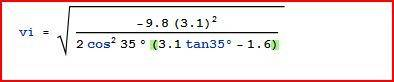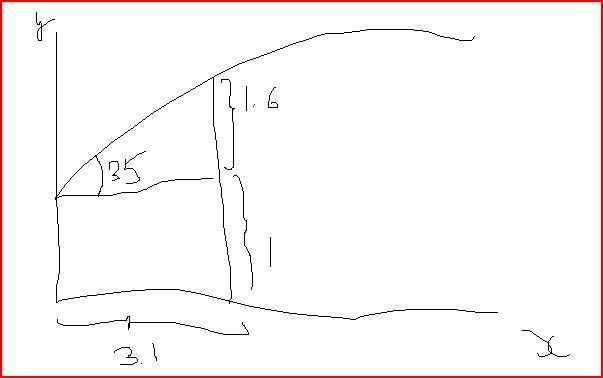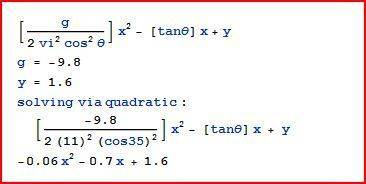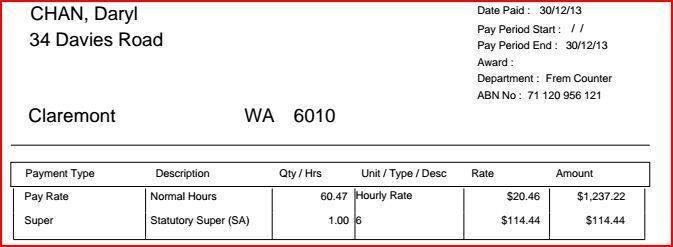# Projectile trajectories

## Homework Statement

A construction worker stands in a 2.6m deep hole, 3.1m from the edge of the hole. He tosses the hammer to a person outside the hole. If the hammer leaves his hand 1m above the bottom of the hole at an angle of 35, what is the minimum speed needed to clear the edge.

## The Attempt at a SolutionWhy does the book states the answer = 11m/s?

This is really frustrating!
I am getting 7.8......

Last edited:

SteamKing
Staff Emeritus
Homework Helper
Draw a sketch of the problem. This may help you setting up the equations of motion. You should be able to determine the book's solution.

BTW, you can't arbitrarily ignore the negative sign under the square root.

mfb
Mentor
35° relative to the horizontal?
Where does your formula come from? It gives a wrong result.

@SteamKing: the sign is fine, the subtraction in the denominator cancels it.

Draw a sketch of the problem. This may help you setting up the equations of motion. You should be able to determine the book's solution.

BTW, you can't arbitrarily ignore the negative sign under the square root.

The book has given the sketch.

In fact, the book has given the equation(in symbolic expression) and stated the answer is 11m/s but upon substituting the x and y coordinatate of 3.1m and 1.6m respectively, I don't arrive at 11m/s.
The book has given a sketch too.

35° relative to the horizontal?
Where does your formula come from? It gives a wrong result.

@steamking: The sign is fine, the subtraction in the denominator cancels it.35° relative to the horizontal?
Where does your formula come from? It gives a wrong result.

@SteamKing: the sign is fine, the subtraction in the denominator cancels it.

It comes from t = x/vi cos theta

y = vi sin theta . t - 0.5g t^2

sub t into y-coordinate to get y as a function of x-coordinate.
Once the equation is derived, sub x= 3.1 and y = 1.6
but it doesn't give me 11m/s if I express it in terms of vi

mfb
Mentor
I can confirm the book's answer. You did something wrong at solving the equation for the velocity.

SteamKing
Staff Emeritus
Homework Helper
35° relative to the horizontal?
Where does your formula come from? It gives a wrong result.

@SteamKing: the sign is fine, the subtraction in the denominator cancels it.

I suggest you check your calculations again.

(3.1 tan 35) - 1.6 > 0

mfb
Mentor
Oh sorry, for some reason I squared the tan instead of the cos.

I suggest you check your calculations again.

(3.1 tan 35) - 1.6 > 0

I basically run the calculation of the workings in my OP but it doesn't turn up to be 11m/s

mfb
Mentor
The approach of post #6 is right, the formula in post #1 is not. If you need help to find your error, show the steps you made in between.

The approach of post #6 is right, the formula in post #1 is not. If you need help to find your error, show the steps you made in between.

Post 1 is nothing more than a mathematical expression of post 6.

I'm sure post 1 is right. I think the issue might be that on my calculator I did (2cos35)^2 instead of 2cos(35)^2

I'm getting 10.036m/s

x = vcos(theta).t
y = vsin(theta).t - 0.5gt^2

t = x/(vcos(theta))

y = vsin(theta)[(x)/vcos(theta)] - 0.5g[(x)/vcos(theta)]^2

y = xtan(theta) - [(g)/(2v^2 (cos(35)^2))

v = SQRT[(gx^2)/(2cos(theta)^2(xtan(theta)-y))

Sub x = 3.1 and y = 1.6
g = -9.8

It's giving me 10m/s and not 11m/s

mfb
Mentor
You are using the wrong sign for g. Use a negative sign in the equation for y=... or for g itself, but not both, otherwise you are accelerating upwards.

That cannot be the only error (check -> 12.5), but I don't see the error right now.

You are using the wrong sign for g. Use a negative sign in the equation for y=... or for g itself, but not both, otherwise you are accelerating upwards.

That cannot be the only error (check -> 12.5), but I don't see the error right now.

I don't see any other error aside from the negative g that I use. And why shouldn't g be negative? It has always been negative according to convention

You are using the wrong sign for g. Use a negative sign in the equation for y=... or for g itself, but not both, otherwise you are accelerating upwards.

That cannot be the only error (check -> 12.5), but I don't see the error right now.

Besides, you're not getting 11m/s too. The link from you shows wolfram getting 12m/s+

You are using the wrong sign for g. Use a negative sign in the equation for y=... or for g itself, but not both, otherwise you are accelerating upwards.

That cannot be the only error (check -> 12.5), but I don't see the error right now.

Check the jpg in my op. -9.8 in the working indicates a negative g.

I have found v to be 11m/s.

To find where the hammer lands:This is a very juvenile question to ask but is the a, b and c values correct?
Solving via quadratic, I'm not getting x= 3.1 or x = 8.7.
It's a very simple problem but this question is taking me more than 1 day to solve!!!!

mfb
Mentor
I don't see any other error aside from the negative g that I use. And why shouldn't g be negative? It has always been negative according to convention
Okay, but if g is negative (so y is pointing upwards), the equation of motion is ##y=v_y t + \frac{1}{2} g t^2## and not minus.

Besides, you're not getting 11m/s too. The link from you shows wolfram getting 12m/s+
That's why I said "this cannot be the only error". Check the WolframAlpha link in post 7, 11m/s is right.

What do you get for x with the reverse attempt? Keep in mind that 11m/s is not the exact value.

Okay, but if g is negative (so y is pointing upwards), the equation of motion is ##y=v_y t + \frac{1}{2} g t^2## and not minus.

That's why I said "this cannot be the only error". Check the WolframAlpha link in post 7, 11m/s is right.

What do you get for x with the reverse attempt? Keep in mind that 11m/s is not the exact value.

I have gotten 11m/s

Okay, but if g is negative (so y is pointing upwards), the equation of motion is ##y=v_y t + \frac{1}{2} g t^2## and not minus.

That's why I said "this cannot be the only error". Check the WolframAlpha link in post 7, 11m/s is right.

What do you get for x with the reverse attempt? Keep in mind that 11m/s is not the exact value.I have found v to be 11m/s.

To find where the hammer lands:

This is a very juvenile question to ask but is the a, b and c values correct?
Solving via quadratic, I'm not getting x= 3.1 or x = 8.7 but these 2 values are what the book states.